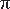Tapping into mathematics

Start this free course now. Just create an account and sign in. Enrol and complete the course for a free statement of participation or digital badge if available.

Free course

# Learning outcomes

By the end of this free course you should have:

• started to become familiar with your scientific or graphics calculator and its use in investigating everyday problems;

• developed good practice in relation to calculator use;

• be able to use your calculator for the following:

• arithmetic involving the operations of +, −, ×, ÷ and the use of brackets;

• squaring and square rooting numbers;

• reciprocals and powers of numbers;

• calculations involving percentages, large and small numbers, scientific notation and;

• be able to understand the concepts of a mathematical function and its inverse (doing and undoing), rounding answers appropriately, fixing the number of decimal places displayed by a calculator, storing numbers in a calculator memory.

MU120_4MCAL